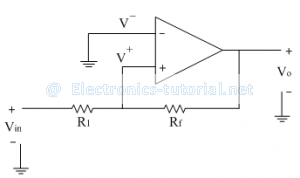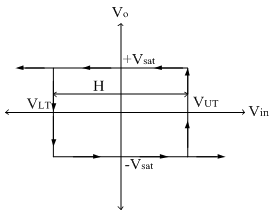Home > analog integrated circuits > schmitt trigger > non inverting schmitt trigger

# Non-inverting Schmitt Trigger

In non-inverting Schmitt trigger, the input signal is applied at the non-inverting terminal of op-amp as shown below.In this the voltage present at non-inverting terminal (V+) is compared with the voltage present at inverting terminal (V- = 0V)
The operation of the circuit can be explained with the help of two conditions:
1. When V^+>V^- outputvoltage Vo=+V_sat
2. When V^+<V^- outputvoltage Vo=-V_sat
In this circuit, two voltages Vin and Vo are acting simultaneously at a time. By using superposition theorem we have to calculate the value of voltage present at non-inverting terminal i. e. V+
When Vo is shorted to ground
V^+= [Vin/(R1+Rf )] Rf
When Vin is shorted to ground
V^+=[Vo/(R1+Rf )] R1
Thus total voltage V+ is given as
∴ V^+=[Rf/(R1+Rf )] Vin+[R1/(R1+Rf )] Vo
Equation of triggering points:
Let output is in positive saturation ∴Vo=+Vsat
∴V^+=[Rf/(R1+Rf )] Vin+[R1/(R1+Rf )](+Vsat )
Now here output is changing state to +Vsat when V+ crosses V- = 0V. While changing the state input voltage is crossing the threshold point i.e.VT.

Thus at the time of state change we can have
Vin = VT and V+ = 0V.
Substituting the above values in equation of V+, we get triggering point as follows.
∴0=[Rf/(R1+Rf )] VT+[R1/(R1+Rf )](+Vsat )
∴[Rf/(R1+Rf )] VT=-[R1/(R1+Rf )](+Vsat )
∴VT=-[R1/(R1+Rf )][(R1+Rf)/Rf ](+Vsat )
∴VT=-[R1/Rf ](+Vsat ) ……referred as lower threshold point V_LT
Similarly when output is in –Vsat∴Vo=-Vsat
∴V^+=[Rf/(R1+Rf )] Vin+[R1/(R1+Rf )](-Vsat )
Now here also output is changing state to -Vsat when V+ crosses V- = 0V. While changing the state input voltage is crossing the threshold point i.e. VT. Thus at the time of state change we can have
Vin = VT and V+ = 0V.
Substituting the above values in equation of V+, we get triggering point as follows.
∴0=[Rf/(R1+Rf )] VT+[R1/(R1+Rf )](-Vsat )
∴[Rf/(R1+Rf )] VT=-[R1/(R1+Rf )](-Vsat )
∴VT=[R1/(R1+Rf )][(R1+Rf)/Rf ](+Vsat )
∴VT=[R1/Rf ](+Vsat )……referred as upper threshold point V_UT
The transfer characteristics are shown below.The hysteresis width can be calculated as
H=VUT-VLT
∴H= R1/Rf (+Vsat )-[-R1/Rf (+Vsat ) ]
∴H=2R1/Rf (Vsat )